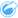# Calculate Ratio

Calculate Ratio uses a simple division equation to determine the relationship between two number variables. Calculating ratios is a form of normalization, which makes it especially useful for comparing areas that are not uniform in size or population.

##### Note:

You can also calculate a ratio using the data table.

## Example

A business analyst for a consortium of colleges wants to know which states have colleges with the highest return on investment. Calculate Ratio can be used to create a ratio between the average earnings after graduation and the average cost of college.

## Use the Calculate Ratio capability

Use the following steps to run the Calculate Ratio analysis capability:

1. Create a map, chart, or table using the dataset with which you want to calculate a ratio.
2. Click the Action button.
3. Do one of the following:
• If your card is a chart or table, click How is it related? in the Analytics pane.
• If your card is a map, click the Find answers tab and click How is it related?.
4. Click Calculate Ratio.
5. For Choose a layer, select the dataset with which you want to calculate a ratio.
6. For Choose number fields, choose the fields you want to use for the numerator and denominator. The fields must be a number or rate/ratio.
7. For Name the result field, enter a name for the new field.
8. Click Run.

A new rate/ratio field is added in the chosen dataset.

## Usage notes

Calculate Ratio can be found using the Action buttonunder How is it related? in the Find answers tab. The input layer can be a point, line, or area layer.

Two number fields must be chosen as the numerator and the denominator:

• Numerator—The number that will be divided in a ratio. In the case of average household income, total income would be the numerator.
• Denominator—The number that will be used to divide the numerator in a ratio. In the case of per capita crime rate, total population would be the denominator.

Calculate Ratio adds a new rate/ratio field to the input layer. You must include a new field name to run the calculation.